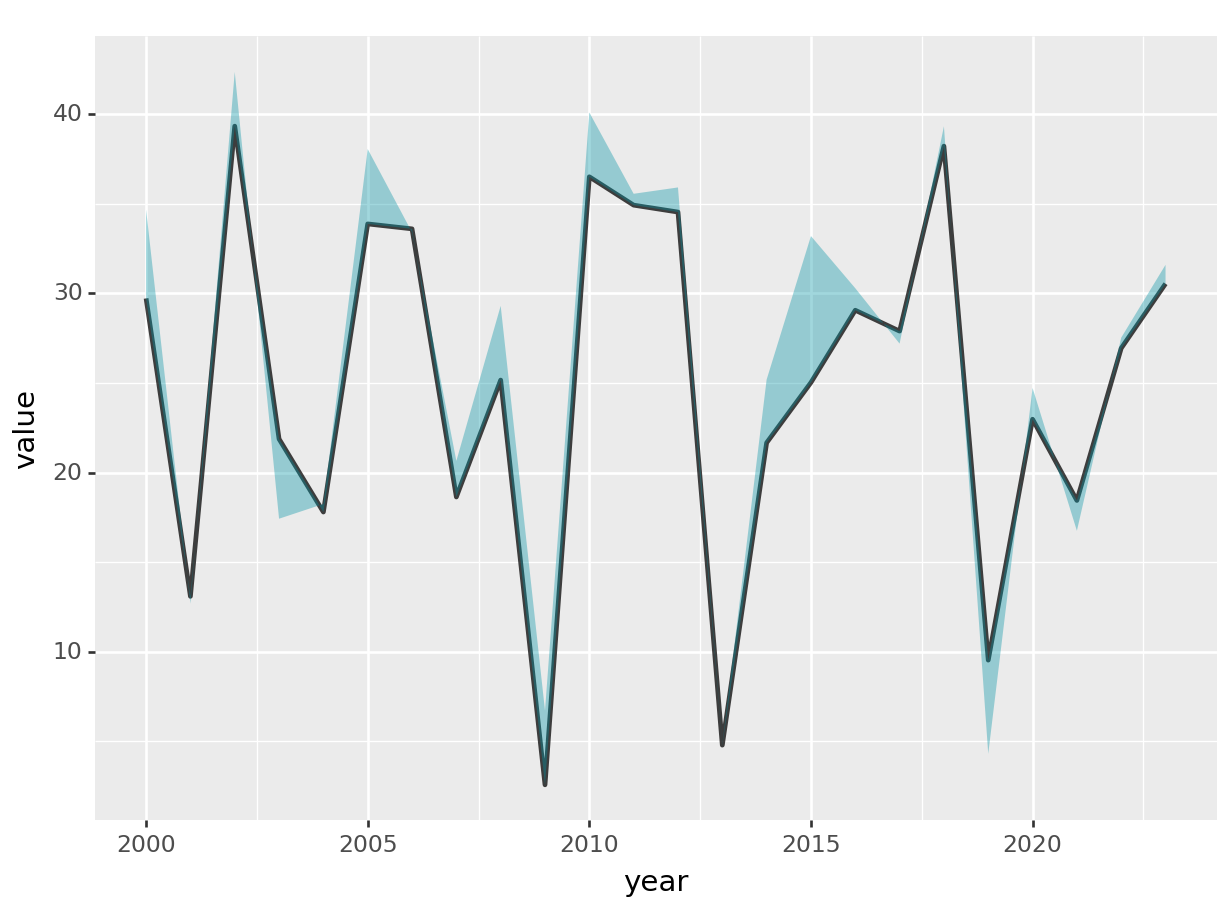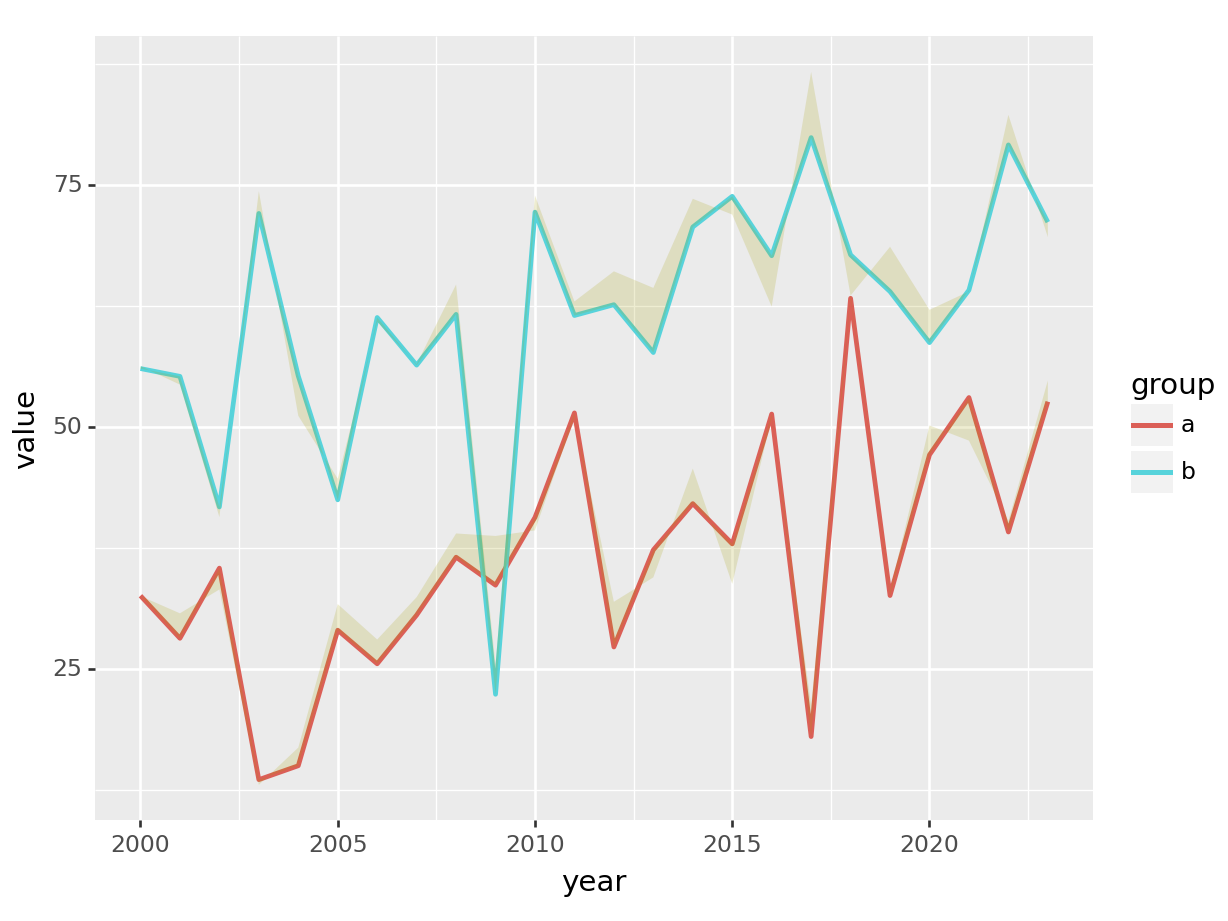Plot your confidence interval easily with Python! With plotnine geom_ribbon() you can add shadowed areas to your lines. We show you how to deal with it!After the high interest rate of our GGplot shadowing confidence interval with R post, we are sharing as easy as that how to do it properly in Python `Plotnine` module.

It’s not a trivial issue as long as you need to melt your data in order to achieve a tidy (R tidiverse concept) format. In Python you can easily achieve it both with Pandas and Polars. Once you have this format in your data frame, all you need is to call geom_ribbon().

## Plotnine geom_ribbon with Polars dataframe

``````from plotnine import *
import polars as pl
import numpy as np

np.random.seed(1234)

df = pl.DataFrame({
"year": range(2000, 2024),
"value": [np.random.normal(25, 10) for i in range(24)],
"noise": [np.random.normal(1,3) for i in range(24)],
})

(
ggplot(
data=df
) + geom_line(aes(x="year", y="value"), color = "#000000bd", size = 1)
+ geom_ribbon(aes(x="year", ymin = "value", ymax = "value + noise"), fill="#0294a55e")
)``````
``## <Figure Size: (640 x 480)>``For a multi-line plot in Python Plotnine, yout should include the `group` and `colour` aesthetic as follows:

``````d2f = pl.DataFrame({
"year": list(range(2000, 2024))*2,
"group": np.sort(['a', 'b']*24),
"value": [np.random.normal(i+25, 10) for i in range(24*2)],
"noise": [np.random.normal(1,3) for i in range(24*2)],
})

(
ggplot(
data=d2f
) + geom_line(aes(x="year", y="value", group="group", colour="group"), size = 1)
+ geom_ribbon(aes(x="year", ymin = "value", ymax = "value + noise", group="group"), fill="#a59e022e")
)``````
``## <Figure Size: (640 x 480)>``